### Home > CA > Chapter Ch12 > Lesson 12.1.1 > Problem12-7

12-7.
1. Simplify each expression below. Your answer should contain no parentheses and no negative exponents. Homework Help ✎

1.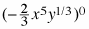2. (251/2x5)(4x−6)

3. 5t−3

4.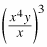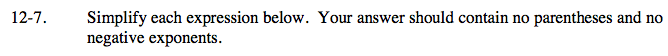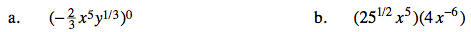Anything to the zero power equals 1.

When multiplying like bases, add their exponents.

20x −1 = ?

$\frac{20}{x}$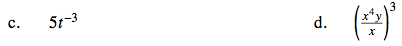See the 'step' in part (b).

Rewrite the expression as multiplication three times.
Then follow the steps in part (b).